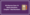# RD Sharma Class 10 Maths Solutions Chapter 1 Real NumbersAre You Facing Difficulty Solving Class 10 Maths Chapter – 1 Real Numbers Problems? Here are the Solutions for it. ie.  RD Sharma Class 10 Maths Solutions, Which are Free downloadable in PDF format to Boost Class 10 Board Exams with Ease. All the Exercise Questions are Listed in RD Sharma Class 10 Maths Solutions Chapter 1 Real Numbers.

## RD Sharma Class 10 Maths Solutions Real Numbers Chapter -1

The Class 10 Maths Real Numbers Chapter 1 Solutions are Prepared by Subject Experts according to CBSE NCERT Curriculum.

Download Complete RD Sharma Class 10 Solutions PDF with all Chapter Listed to make your Exam preparation Easier.

### Class 10 Maths Chapter-1 Real Numbers

In CBSE Class 10, you began your exploration of the world of real numbers and encountered irrational numbers. let’s know the importance of Real Numbers in this blog.

There are two very important properties of positive integers

• Euclid’s division algorithm
• Fundamental Theorem of Arithmetic.

Euclid’s division algorithm, as the name suggests, has to do with divisibility of integers. S tated simply, it says any positive integer a can be divided by another positive integer b in such a way that it leaves a remainder r that is smaller than b. Many of you probably recognise this as the usual long division process. Although this result is quite easy to state and understand, it has many applications related to the divisibility properties of integers. We touch upon a few of them, and use it mainly to compute the HCF of two positive integers.

The Fundamental Theorem of Arithmetic, on the other hand, has to do something with multiplication of positive integers. You already know that every composite number can be expressed as a product of primes in a unique way — this important fact is the Fundamental Theorem of Arithmetic.

### Benefits of Class 10 Maths RD Sharma Chapter 1 Solutions

• Helps students to understand and solve Problems in a Detailed Manner
• The Effectives of their Explanations to the Problems lasts long in Students Mind
• RD Sharma class 10 Maths Solutions Book is enough to score more marks in the Exam